# ICSE Class 10 Mathematics 2022 Semester-1 Sample Paper

There are three sections in the paper. Maximum Marks are 40 and Time allowed is one and a half hours. All questions are compulsory.

### Section A [16 Marks]

1. If matrix A is of order 3 x 2 and matrix B is of order 2 x 2 then the matrix AB is of order

1. 3 x 2
2. 3 x 1
3. 2 x 3
4. 1 x 3

2. The percentage share of SGST of total GST for an Intra-State sale of an article is

1. 25%
2. 50%
3. 75%
4. 100%

3. ABCD is a trapezium with AB parallel to DC.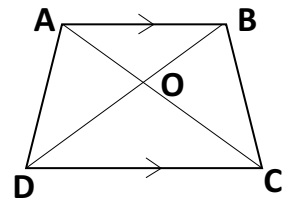Then the triangle similar to ΔAOB is

2. ΔACB
3. ΔCOD
4. ΔCOB

4. The mean proportion between 9 and 16 is

1. 25
2. 144
3. 12

5. A man deposited ₹500 per month for 6 months and received ₹3300 as the maturity value. The interest received by him is:

1. 1950
2. 300
3. 2800
4. none of these

6. The solution set representing the following number line is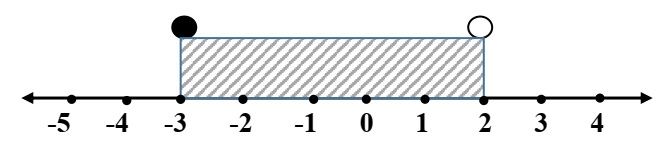1. {x: x ∈ R, -3 ≤ x < 2}
2. {x: x ∈ R, -3 < x < 2}
3. {x: x ∈ R, -3 < x ≤ 2}
4. {x: x ∈ R, -3 ≤ x ≤2}

7. The first three terms of an arithmetic progression (A. P.) are 1, 9, 17, then the next two terms are

1. 25 and 35
2. 27 and 37
3. 25 and 33
4. none of these

8. If ΔABC ~ ΔQPR then the corresponding proportional sides are

1. AB/QR = BC/RP
2. AC/QR = BC/RP
3. AB/QR = BC/QP
4. AB/PQ = BC/RP

9. If x ∈ 𝑊 , then the solution set of the inequation -x > -7, is

1. {8,9,10…}
2. {0,1,2,3,4,5,6}
3. {0,1,2,3 …}
4. { -8, -9, -10…}

10. The roots of the quadratic equation 4𝑥2 - 7x + 2 = 0 are 1.390, 0.359.The roots correct to 2 significant figures are

1. 1.39 and 0.36
2. 1.3 and 0.35
3. 1.4 and 0.36
4. 1.390 and 0.360

11. 1.5, 3, x and 8 are in proportion, then x is equal to

1. 6
2. 4.5
3. 16

12. If a polynomial 2𝑥2 - 7x - 1 is divided by (x + 3), then the remainder is

1. -4
2. 38
3. -3
4. 2

13. If 73 is the nth term of the arithmetic progression 3, 8, 13, 18…, then ‘n’ is

1. 13
2. 14
3. 15
4. 16

14. The roots of the quadratic equation x2 + 2x + 1 = 0 are

1. Real and distinct
2. Real and equal
3. Distinct
4. Not real / imaginary

15. Which of the following statement is not true?

1. All identity matrices are square matrix
2. All null matrices are square matrix
3. For a square matrix number of rows is equal to the number of columns
4. A square matrix all of whose elements except those in the leading diagonal are zero is the diagonal matrix

16. If (x - 2) is a factor of the polynomial x3 + 2x2 - 13x + k, then ‘k’ is equal to

1. -10
2. 26
3. -26
4. 10

### Section B [12 Marks]

17. A man deposited ₹1200 in a recurring deposit account for 1 year at 5% per annum simple interest. The interest earned by him on maturity is

1. 14790
2. 390
3. 4680
4. 780

18. If x2 - 4 is a factor of polynomial x3+ x2 - 4x - 4, then its factors are

1. (x-2) (x+2) (x+1)
2. (x-2) (x+2) (x-1)
3. (x-2) (x-2) (x+1)
4. (x-2) (x-2) (x-1)

19. The following bill shows the GST rates and the marked price of articles A and B: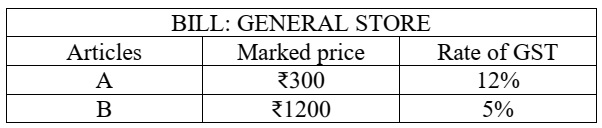The total amount to be paid for the above bill is:

1. 1548
2. 1596
3. 1560
4. 1536

20. The solution set for the linear inequation -8 ≤ x - 7 < -4, x ∈ 𝐼 is

1. {x: x ∈ R, -1≤ x < 3}
2. {0, 1, 2, 3}
3. {-1, 0, 1, 2, 3}
4. { -1, 0, 1, 2}

21. If 5a/7b = 4c/3d, then by Componendo and dividendo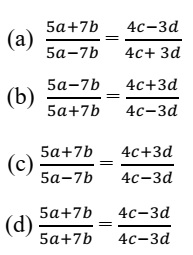22. If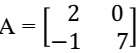then A2 is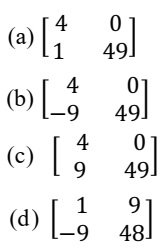### Section C [12 Marks]

23. The distance between station A and B by road is 240 km and by train it is 300 km. A car starts from station A with a speed x km/hr whereas a train starts from station B with a speed 20 km/hr more than the speed of the car.

(i) The time taken by car to reach station B is

1. 240/x
2. 300/x
3. 20/x
4. 300/(x+2)

(ii) The time taken by train to reach station A

1. 240/x
2. 300/x
3. 20/x
4. 300/(x+2)

(iii) If the time taken by train is 1 hour less than that taken by the car, then the quadratic equation formed is

1. x2 + 80x - 6000 = 0
2. x2 + 80x - 4800 = 0
3. x2 + 240x - 1600 = 0
4. x2 -80x + 4800 = 0

(iv) The speed of the car is

1. 60 km/hr
2. 120 km/hr
3. 40 km/hr
4. 80 km/hr

24. In the given triangle PQR, AB || QR, QP || CB and AR intersects CB at O.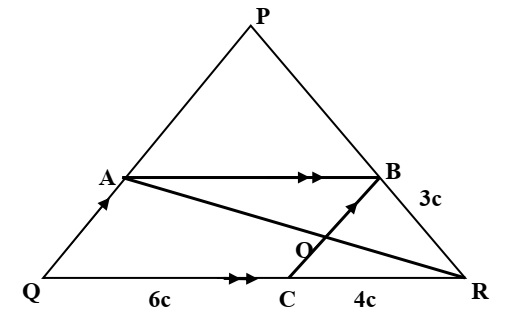Using the given diagram answer the following question:

(i) The triangle similar to ΔARQ is

1. ΔORC
2. ΔARP
3. ΔOBR
4. ΔQRP

(ii) ΔPQR ~ΔBCR by axiom

1. SAS
2. AAA
3. SSS
4. AAS

(iii) If QC = 6 cm, CR = 4 cm, BR = 3 cm. The length of RP is

1. 4.5 cm
2. 8 cm
3. 7.5 cm
4. 5 cm

(iv) The ratio PQ : BC is

1. 2 : 3
2. 3 : 2
3. 5 : 2
4. 2 : 5

25. The nth term of an arithmetic progression (A.P.) is (3n + 1)

(i) The first three terms of this A. P. are

1. 5, 6, 7
2. 3, 6, 9
3. 1, 4, 7
4. 4, 7, 10

(ii) The common difference of the A.P. is

1. -3
2. 2

(iii) Which of the following is not a term of this A.P.

1. 25
2. 27
3. 28
4. 31

(iv) Sum of the first 10 terms of this A.P. is

1. 350
2. 175
3. -95
4. 70